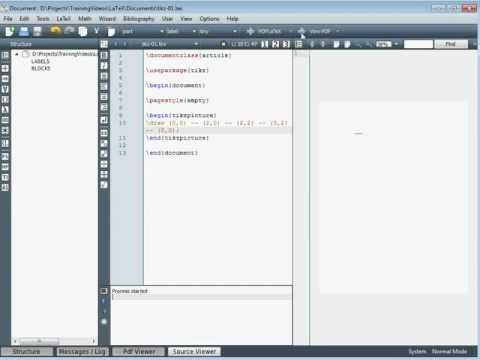# Posts Tagged ‘ graph ’

## Timeline graph with ggplot2

July 7, 2012
ByThis post shows how to create a timeline graph by using ggplot2. Let’s start by loading the ggplot2 library. Next let’s create a dataset which we will use to feed the graph. In the last column (y), I create random positive values for the first three rows (which will be  Read more »The post Timeline graph with ggplot2 appeared...

## igraph and SNA: an amateur’s dabbling

June 29, 2012
ByI’ve been playing with the igraph package a bit lately (see previous post HERE) and wanted to approach a problem I once visited in the past. The basic gist of the problem is this: Students in a class are asked … Continue reading →

## igraph and structured text exploration

June 29, 2012
ByI am in the slow process of developing a package to bridge structured text formats (i.e. classroom transcripts)  with the tons of great R packages that visualize and analyze quantitative data (If you care to play with a rough build … Continue reading →

## Tikz Introduction

September 27, 2011
ByThe pgf drawing package for LaTeX provides facilities for drawing simple of complicated pictures within a LaTeX document. There are many options available within the package and in this post we consider some of the basics to get up and running. Fast Tube by Casper As with all LaTeX documents we need to select a

## gridExtra – Multiple plots from ggplot2

June 8, 2011
ByThanks to this great post http://www.imachordata.com/?p=730 we can now put multiple plots on a display with ggplot2. This provides somewhat similar functionality to ‘par(mfrow=c(x,y))’ which would allow multiple plots with the base plot function. gridExtra doesn’t have quite the same level of options as ‘par’, but the syntax is simple. grid.arrange( graph1, graph2, ncol=2 Simple. ‘grid.table’

## Graph Bisection in R

April 14, 2011
ByRecently I had to partition a set of SNPs into a training set and a test set. Making a random split would not do: both sets would likely contain very similar SNPs due to linkage disequilibrium (LD), making them non-independent. … Continue reading →

## Le Monde puzzle #13

April 13, 2011
ByThis week, Le Monde offers not one but three related puzzles: Is it possible to label the twelve edges of a cube by consecutive numbers such that the sum of the edge numbers at any of the eight nodes is constant? Is it possible to label the eight nodes of a cube by consecutive numbers

## Graphing – margins, titles, mtext, workspace

February 21, 2011
ByThis is a great post, very true, not enough of R’s graphics are well displayed online to really see how to achieve what the often ambiguous ‘help’ information suggests. http://research.stowers-institute.org/efg/R/Graphics/Basics/mar-oma/index.htm I particularly find “mtext(“lol”, outer=T)” to be particularly useful (requires “oma=c(2,2,2,2)” or similar). http://addictedtor.free.fr/graphiques/ This site is somewhat of the way there, but I’ve found

## Graph gallery in R

January 6, 2011
ByR is sometime criticized for producing graphs not as elaborated as Matlab ones, or other softwares’. Here is a link to a graph gallery by Romain François to “enhance your data visualization with R”. The corresponding R code is given. Might be useful for ENSAE students for ‘statap’ projects. Below are four examples. The maps

## Le Monde puzzle 

December 1, 2010
By$Le Monde puzzle $

This week(end), the Le Monde puzzle can be (re)written as follows (even though it is presented as a graph problem): Given a square 327×327 symmetric matrix A, where each non-diagonal entry is in {1,2,3,4,5} and , does there exist a triplet (i,j,k) such that Solving this problem in R is very easy. We can create